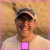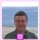# Is it possible to pass/return structs by value from EXTERN functions?For example:

```// c lib
struct vec2 { float x, y; };
vec2 normalized(vec2 v);

// forth
LIBRARY: c-lib.dll

BEGIN-STRUCTURE vec2
CELL FIELD x
CELL FIELD y
END-STRUCTURE

EXTERN: vec2 normalized( vec2 v );```

The above doesn't work (as-is), obviously, but wasn't sure if there was a way to do this or not?by Stephen Pelc (1.5k points)
`EXTERN: void * normalized( void * pv );`